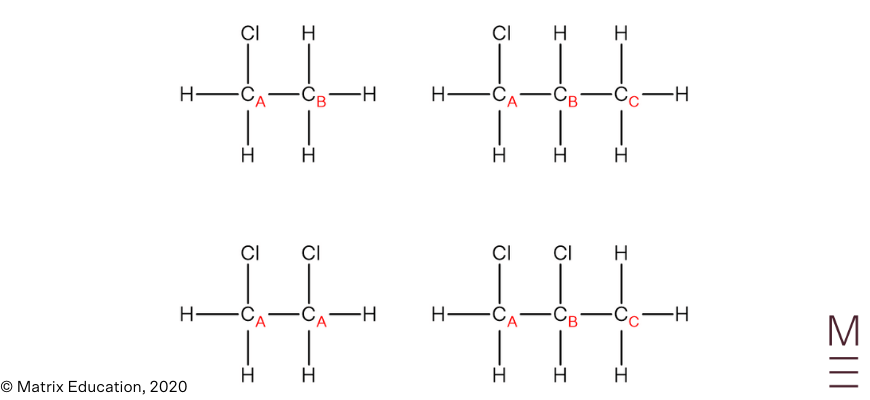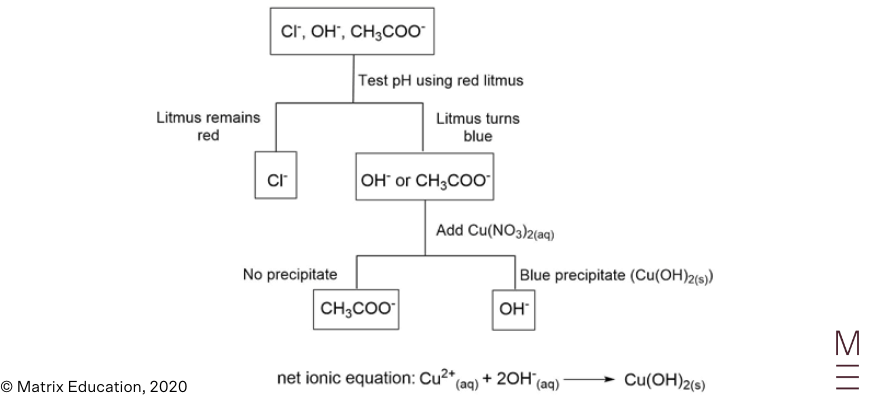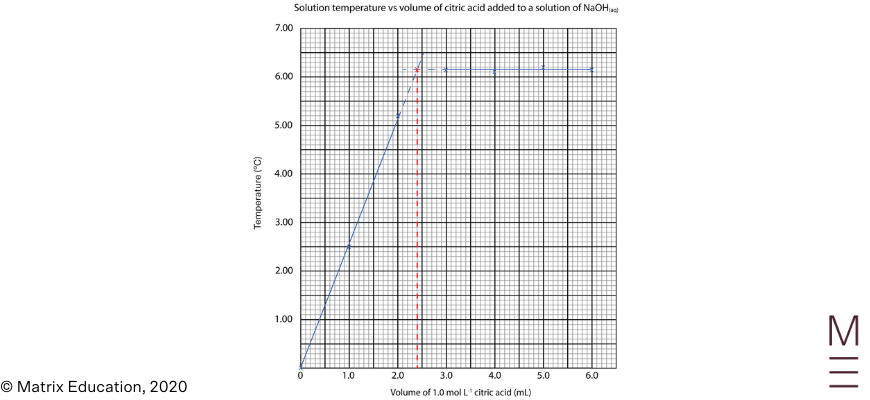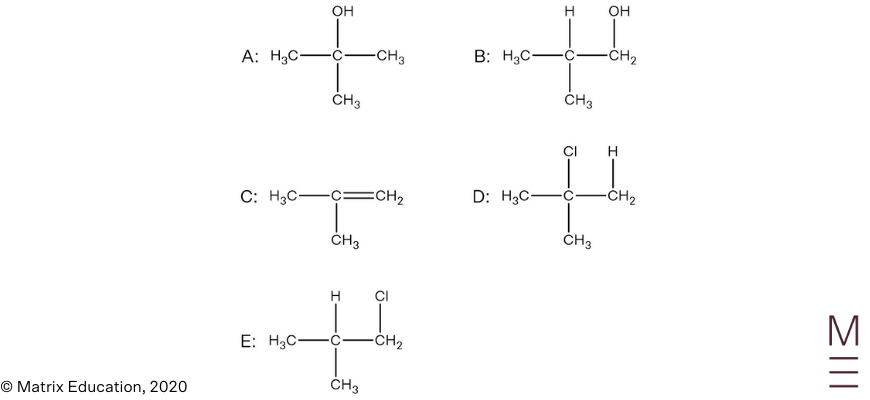# Chemistry Exam Paper Worked Solutions

Keen to see how you went in the 2020 HSC Chemistry exam? Well, our Chemistry team have been hard at work cooking up the solutions for you!

Below are the answers and worked solutions to this year’s Chemistry HSC Exam.

## Section 1: Multiple Choice Solutions

 Question Solution Explanation 1 B Charged particles travel along a curved path in a magnetic field 2 C Phenol red will be yellow at pH 5 (for face cleanser) and red at pH 9 (for soap). All other indicators will show the same colour for both the face cleanser and the soap. 3 C Alkanes, alcohols and esters are neutral. Amines are basic. 4 A Infrared spectroscopy identifies different types of bonds. Butane and propane both only have C-C and C-H bonds. 5 A Two signals in the spectrum indicate the molecule only has two carbon environments. Chloroethane has two carbon environments. 1-chloropropane has 3 carbon environments, 1,2-dichloroethane has 1 carbon environment and 1,2-dichloropropane has 3 carbon environments.6 D Hydroxyl group has highest priority, therefore carbon bearing the OH group will have the lowest number, and the chain is numbered right to left.The substituents are named in alphabetical order, hence bromo is named before fluoro. 7 C Functional group isomers result when the atoms in the molecules are arranged to form different functional groups. Compound 2 and compound 3 have different functional groups. Chain isomers involve rearrangement of the carbons in the backbone. Compounds 1 and 2 and compounds 1 and 4 have the same carbon chain (4 carbons in the backbone) therefore are not chain isomers. Position isomers result when molecules have the same carbon chain but the functional group is at a different location. Compounds 2 and 4 do not have the same functional groups. 8 D A solution is most effective as a buffer at the pH corresponding to the half-equivalence point. From the titration curve, this occurs at pH 9. 9 A The compound in A is a carboxylic acid, which reacts readily with sodium hydrogen carbonate (a base). The other compounds are not acidic: compound in B is a ketone and compound in C and D are alcohols. 10 D $$NaCl$$ is a neutral salt, $$NH_4Cl$$ is an acidic salt and $$NaCH_3COO$$ is a basic salt. From least to most acidic (basic → neutral → acidic): $$NaCH_3COO, NaCl, NH_4Cl$$ 11 B Mixing solutions of $$Ba(OH)_2$$ and $$MgSO_4$$ results in the formation of the precipitates $$BaSO_{4(s)}$$ and $$Mg(OH)_{2(s)}$$. Each of the other multiple choice options only produces one precipitate. 12 C The softening of plastics occurs due to a weakening of the intermolecular forces between polymer chains. Based on the portion of the polymer shown, the intermolecular forces that are present are dipole-dipole forces and dispersion forces. (hydrogen bonding cannot occur as there is no N-H, O-H, or F-H bond). 13 B The conversion of methanoic acid to methanol results in the VSEPR shape around the carbon atom changing from trigonal planar to tetrahedral.14 B $$K_w = [H_3O^+][OH^-] = 5.5 \times 10^{-14}$$ $$[H_3O^+] = [OH^-] = \sqrt{K_w} = 2.34 \times 10^{-7}$$ $$\text{pH} = \text{-log}_{10}[H_3O^+] = 6.63$$ 15 D Option A cannot produce a peak in the mass spectrum as it is uncharged. Option B has an m/z of 16. Option C contains 4 carbon atoms in the fragment, while chloroacetamide only contains 2 carbon atoms. 16 C The yield increases with temperature indicating that the forward reaction is endothermic. The yield decreases with pressure, indicating that the product side of the equation contains more moles of gas than the reactant side. 17 D The following equilibrium occurs in the saturated solution of copper(II) sulfate : $$CuSO_{4(s)} ⇌ Cu^{2+}_{(aq)} + SO_4^{2-}$$$$_{(aq)}$$ Addition of sodium hydroxide results in the precipitation of $$Cu(OH)_{2(s)}$$, which reduces the concentration of $$Cu^{2+}_{(aq)}$$ and disturbs the equilibrium. As a result, more $$CuSO_{4(s)}$$ will dissolve to minimise the disturbance (Le Chatelier’s Principle). The blue colour will fade as the concentration of $$Cu^{2+}$$ ions in the final solution is lower than in the original solution. 18 A $$HCO_3^-$$ is amphiprotic, meaning that it can act as either an acid or base. As the pH of the solution is greater than 7, $$HCO_3^-$$ is acting as a base and accepts a proton from water. This indicates that water is a stronger acid than $$HCO_3^-$$. 19 D Cooling the system causes a reduction in the forward and reverse reaction rates. As exothermic reactions possess a lower activation energy than endothermic reactions, the forward reaction rate is less affected by cooling than the reverse reaction rate. 20 C $$K_{sp} = [Ag^+]^2[SO_4^{2-}]$$ From the graph, when $$[Ag^+] = 1 \times 10^{-4} \ \text{mol L}^{-1}, [CrO_4^{2-}] = 11 \times 10^{-5} \ \text{mol L}^{-1}$$ Therefore, $$K_{sp} = (1 \times 10^{-4})^2(11 \times 10^{-5}) = 1.1 \times10^{-12}$$

## Section 2: Long response Questions

### Question 21

The molecular ion peak is at $$m/z = 44$$.

Given that the general formula for an alkane is $$C_nH_{2n+2}$$, the alkane is propane $$(C_3H_8)$$

### Question 22

Cation

Perform a flame test:

• Brick red flame indicates $$Ca^{2+}$$
• Apple green flame indicates $$Ba^{2+}$$
• No characteristic flame colour indicates $$Mg^{2+}$$

Anion### Question 23

Temperature: Using temperatures that are beneficial to rate and yield. Optimising temperature can also improve the longevity of catalysts, safety, and cost.

By-products: Creating by-products that are valuable and easy to separate from ethane-1,2-diol.

Availability of reactants: Reactants should be widely available and inexpensive. In this case, oxygen can be obtained from air, and ethene from cracking hydrocarbons.

### Question 24a

The functional group present in both the oil and the biodiesel is an ester.

### Question 24b

 $$C_{14}H_{30}O_{2(l)} + 41/2O_{2(g)} → 14CO_{2(g)} + 15H_2O_{(l)}$$ $$C_{18}H_{38 (l)} + 55/2O_{2(g)} → 18CO_{2(g)} + 19H_{2}O_{(l)}$$

Soot is produced when there is insufficient oxygen for a carbon containing fuel to undergo complete combustion. As biodiesel is more oxygenated than diesel, less $$O_2$$ is required for complete combustion, meaning that soot is less likely to form.

### Question 24c

Energy density of biodiesel in

 $$\text{MJ L}^{-1} = 38\text{ MJ kg}^{-1} \times 0.90\text{ kg L}^{-1} = 34\text{ MJ L}^{-1}$$

Volume of biodiesel required to produce

 $$2141\text{ MJ} = 2141\text{ MJ} / \ 34\text{ MJ L}^{-1} = 63\text{ L (2 s.f.)}$$

### Question 24d

 Advantages Disadvantages Renewable since it can be produced through fermentation of sugar from sugarcane Arable land (which is finite) is required to produce biomass. $$CO_2$$ produced from combustion is recycled through the growth of biomass required for biodiesel Lower energy density meaning more fuel is required to produce the same amount of energy.

### Question 25\begin{align*} n(\text{citric acid}) &= 1.0 \ \text{mol L}^{-1} \times 0.0024 \text{ L} = 0.0024 \ \text{mol} \\ n(NaOH) &= 3 \times n(\text{citric acid}) = 3 \times 0.0024 \ \text{mol} = 0.0072 \ \text{mol in }8.0 \text{ mL} \\ c(NaOH) &= 0.0072 / 0.0080 = 0.90 \ \text{mol L}^{-1} \ \text{(2 s.f.)} \\ \end{align*}

### Question 26a

$$N_{2(g)} + O_{2(g)} ⇌ 2NO_{(g)}$$

### Question 26b

The energy profile diagram shows that the enthalpy of the products is greater than the enthalpy of the reactants indicating that the forward reaction is endothermic and the reverse reaction is exothermic. Moreover, the energy profile diagram shows that the activation energy of the forward reaction is larger than the activation energy for the reverse reaction.

Increasing the temperature increases the average kinetic energy of the collisions, thus a greater proportion of collisions will have energy greater than the activation energy. As a result, increasing the temperature increases both the forward and reverse rates of reaction. However, the percentage increase in the rate of the forward, endothermic reaction is greater than that of the reverse, exothermic reaction. As a result, the concentration of the $$NO$$ increases while the concentration of the reactant $$N_2$$ and $$O_2$$ gases decreases.

As the equilibrium constant ($$K_{eq}$$) for this process is:

$$K_{eq}= \frac{[NO]^2}{[N_2][O_2]}$$

The value of $$K_{eq}$$ will increase with temperature.

### Question 27a### Question 27b

$$K_b= \frac{[C_3 H_7 NH_3^+ ][OH^-]}{[C_3 H_7 NH_2]}$$

 Concentration (mol L-1) $$C_3H_7NH_2$$ $$C_3H_7NH_3^+$$ $$OH^-$$ Initial $$1.00$$ $$0$$ $$0$$ Change $$-x$$ $$+x$$ $$+x$$ Equilibrium $$1.00-x$$ $$x$$ $$x$$

$$K_b = \frac{[x][x]}{[1.00-x]}$$

Assuming $$x$$ is small since $$K_b$$ is small: $$[1.00 – x] ≈[1.00]$$

\begin{align*}
K_b &= \frac{[x][x]}{[1.00]} =4.37 \times 10^{-4} \\
x &=0.0209045 \\
\end{align*}

Therefore, the concentration of hydroxide ions is $$0.0209 \ \text{mol L}^{-1} (\text{3 s.f.})$$

Question 28

Inaccurate as:

• $$NaOH_{(s)}$$ is hygroscopic and therefore not suitable as a primary standard.
• Bromocresol green is used as an indicator. As a weak acid is being titrated by a strong base, the equivalence point would be basic (pH > 7). As a result, bromocresol green will not change colour at the equivalence point.

Unreliable as the results are inconsistent. The experiment should be repeated until enough concordant results have been recorded.

### Question 29### Question 30Infrared spectrum:

Signal between $$1680-1750 \text{ cm}^{-1}$$ indicates the presence of a carbonyl group (C=O). The absence of a broad trough between $$2850-3550 \ \text{ cm}^{-1}$$ indicates that the compound does not contain a hydroxyl group.

$$^{13}\text{C NMR}$$:

The $$^{13}\text{C NMR}$$ spectrum has 4 peaks, indicating there are 4 unique carbon environments, this is consistent with the proposed structure. The peak at 170 ppm is characteristic of the carbonyl carbon and the peak at 70 ppm corresponds to a carbon nucleus adjacent to an oxygen, hence confirms the presence of an ester group.

$$^1\text{H NMR}$$:

The $$^1\text{H NMR}$$ spectrum shows 3 peaks, indicating there are 3 unique proton environments.

The peak at 1.2 ppm corresponds to the protons labelled A. The chemical shift is consistent for alkyl protons, the peak is a doublet which indicates these protons are adjacent to one other non-equivalent proton. Its integration of 6 indicates 6 protons are present in this environment.

The peak at 2.0 ppm corresponds to the protons labelled B. The chemical shift is consistent for protons that are adjacent to a carbonyl group. The singlet indicates there are zero non-equivalent protons on the adjacent carbon atom. An integration of 3 indicates 3 protons are present in this environment.

The peak at 5.0 ppm corresponds to proton labelled C. The chemical shift is consistent for protons that are adjacent to the oxygen of an ester group. The septet indicates there are 6 non-equivalent protons on the adjacent carbon atoms. An integration of 1 indicates 1 proton is present in this environment.### Question 31

Reaction 1: $$Ag^+_{(aq)} + Cl^-_{(aq)} → AgCl_{(s)}$$
Reaction 2: $$Ag^+_{(aq)} + SCN^-_{(aq)} → AgSCN_{(s)}$$

 \begin{align*} n(SCN^-) &= 0.0500 \times 0.02865 = 0.0014325 \ \text{mol} \\ n(Ag^+)_{\text{Reaction 2}}  &= n(SCN^-) = 0.0014325 \ \text{mol} \\ n(Ag^+)_\text{total} = 0.100 \times 0.025 &= 0.0025 \ \text{mol} \\ n(Ag^+)_{\text{Reaction 1}}  &= 0.0025 – 0.0014325 = 0.0010675 \ \text{mol} \\ n(Cl^-) \text{in} \ 100 \text{ mL} &= n(Ag^+)_{\text{Reaction 1}}  = 0.0010675 \ \text{mol} \\ n(Cl^-) \text{in}\ 1 \text{ L} &= 0.010675 \ \text{mol} \\ m(Cl^-) \text{in} \ 1 \text{ L} &= 0.37842875 \text{ g} \\ \end{align*}

Therefore, concentration of $$Cl^- = 378 \text{ mg/L} \text{(3 s.f.)}$$

### Question 32

All three compounds are polar and can form dispersion forces and dipole-dipole forces. Methanol and propanoic acid can also form hydrogen bonding.

However, even though methyl propanoate cannot form hydrogen bonding, it exhibits stronger dispersion forces compared to methanol and propanoic acid due to its larger size. Hence the combined strength of its dispersion forces and dipole-dipole forces are stronger than methanol’s intermolecular forces. Thus, more thermal energy is required to break the intermolecular forces in methyl propanoate to cause boiling.

On the other hand, although propanoic acid has weaker dispersion forces compared to methyl propanoate, propanoic acid has the ability to form two hydrogen bonds per molecule, hence the overall strength of the hydrogen bonds compensates the weaker dispersion forces, allowing propanoic acid to have stronger intermolecular forces compared to methyl propanoate.

### Question 33 a

 \begin{align*} ca(OH)_{2(s)}+ 2HCl_{(aq)} → CaCl_{2(aq)} + 2H_{2}O_{(l)} \\ n(HCl)  = 0.100 x 2.00 = 0.200 \ mol \\ n(Ca(OH) 2 )  = ½ \times n(HCl) = 0.100 \ mol \\ \end{align*}

### Question 33 b

 \begin{align*} n(Ca(OH)_2) \text{in }0.1 \text{ L} &= 0.100 \ \text{mol} \\ c(Ca(OH)_2) &= 0.100 / 0.1 = 1 \ \text{mol/L} \\ \end{align*}

 Concentration (M) $$Ca(OH)_2$$ ⇌ $$Ca^{2+}$$ $$2OH^-$$ Initial – $$1$$ $$0$$ Change – $$+ x$$ $$+ 2x$$ Equilibrium – $$1+x$$ $$2x$$

Since $$K_{sp}$$ is small, assume $$[Ca^{2+}]_{eqm} ≈ [Ca^{2+}]_{\text{initial}} = 1 \text{ M}$$

 \begin{align*} [Ca^{2+}][OH^-]^2 &= 5.02 \times 10^{-6} \\ 1 \times (2?)^2 &= 5.02 \times 10^{-6} \\ 4?^2 &= 5.02 \times 10^{-6} \\ ? &= 0.001120267825 \\ [OH^-] &= 2? = 0.00224053565 \\ pH &= 14 + \text{log}_{10}(0.00224053565) = 11.350 \ (3 \text{s.f.}) \\ \end{align*}

### Question 34

Hydrochloric acid is a strong acid that completely ionises in water:

$$HCl_{(aq)} → H^+_{(aq)} + Cl^-_{(aq)}$$

Hence the $$[H^+] = [HCl]$$

An increase in the concentration of hydrochloric acid by 10 fold results in an increase in the concentration of $$H^+$$ by 10 fold. Since $$pH = \text{-log}_{10}[H^+]$$, each 10 fold increase in $$[H^+]$$ results in a decrease of the pH by 1. Thus the pH decreases incrementally by 1.

Acrylic acid is a weak acid that only partially ionises in water:

$$C_2H_3COOH_{(aq)} ⇌ H^+_{(aq)} + C_2H_3COO^-_{(aq)}$$

Hence the $$[H^+] < [C_2H_3COOH]$$

Thus, an increase in the concentration of acrylic acid by 10 fold will increase the concentration of $$H^+$$ by less than 10 fold. Since $$\text{pH} = \text{-log}_{10}[H^+]$$, this will result in a decrease in the pH by less than 1.
As the concentration of acrylic acid increases, the concentration of all components increase, hence $$Q > K$$. This results in a shift in the reverse direction, which lowers the degree of ionisation. This effectively results in a smaller and smaller change in the $$[H^+]$$ which results in smaller change to the pH as concentration increases.

### Question 35

 \begin{align*} [I_3^- ]_{eq} &= \frac{0.745}{2.76 \times 10^4} = 2.699275 \times 10^{-5} \text{ M} \\ [I_2 ]_{eq} &= \frac{[I^- ]_{\text{initial}} – 3[I_3^- ]_{eq}}{4} = \frac{7.00 \times 10^{-4} – 3 \times (2.699275 × 10^{-5})}{4} = 1.54755 \times 10^{-4} \text{ M} \\ [I^- ]_{eq} &=2 \times [I_2 ]_{eq} =3.09510 \times 10^{-4} \text{ M} \\ K &= \frac{[I_3^-]}{[I^-][I_2]} = \frac{2.699275 \times 10^{-5}}{(3.09510 × 10^{-4})(1.54755 \times 10^{-4})} = 564 \text{ (3 s.f.)} \\ \end{align*}

### Question 36

 \begin{align*} n(NaOH) &= 10.0 / 39.998 = 0.2500125 \ \text{mol} \\ ∆H_{\text{soln}} &= 0.2500125 \times -44.5 = -11.12555628 \ \text{kJ} \\ n(HCl) &= 2.00 \times 0.100 = 0.200 \ \text{mol} \\ \end{align*}

HCl is limiting reactant

 \begin{align*} ∆H_{\text{reaction}} &= 0.200 \times -56.1 = -11.22 \ \text{kJ} \\ ∆H_{\text{total}} &= -11.12555628 + -11.22 = -22.34555628 \text{ kJ} \\ q &= mc∆T \\ q &= 22345.55628 \text{ J} \\ 22345.55628 &= 113 \times 3.99 \times (T_{\text{final}} – 22.5) \\ T_{\text{final}} &= 72.1 \ °\text{C}  \text{ (3 s.f.)} \\ \end{align*}

#### Related Posts

Platform Slot Gacor CMS Checker